## EpsilonsThe capital Greek sigma, that is, repeated addition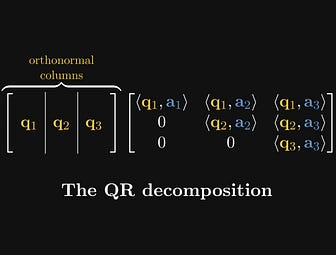matrices + the Gram-Schmidt process = magic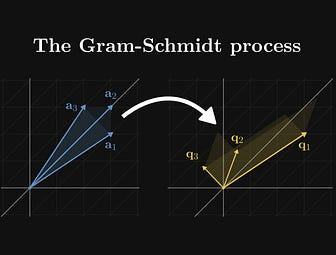Orthogonalizing bases since 1883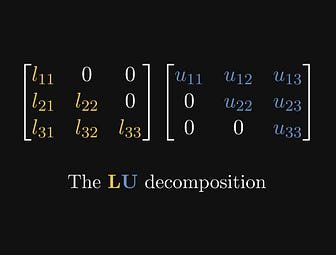How determinants and matrix inverses are calculated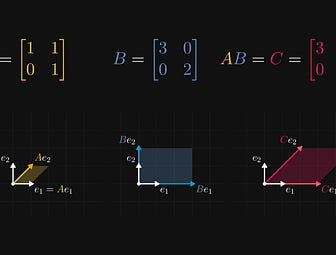The geometric and algebraic interpretation of matrix products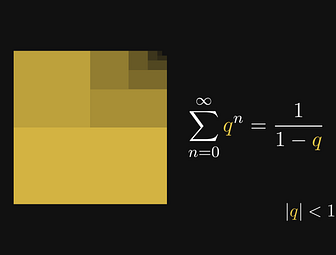One of my favorite formulas is the closed-form of the geometric series.I am amazed by its ubiquity: whether we are solving basic problems or pushing the…